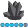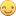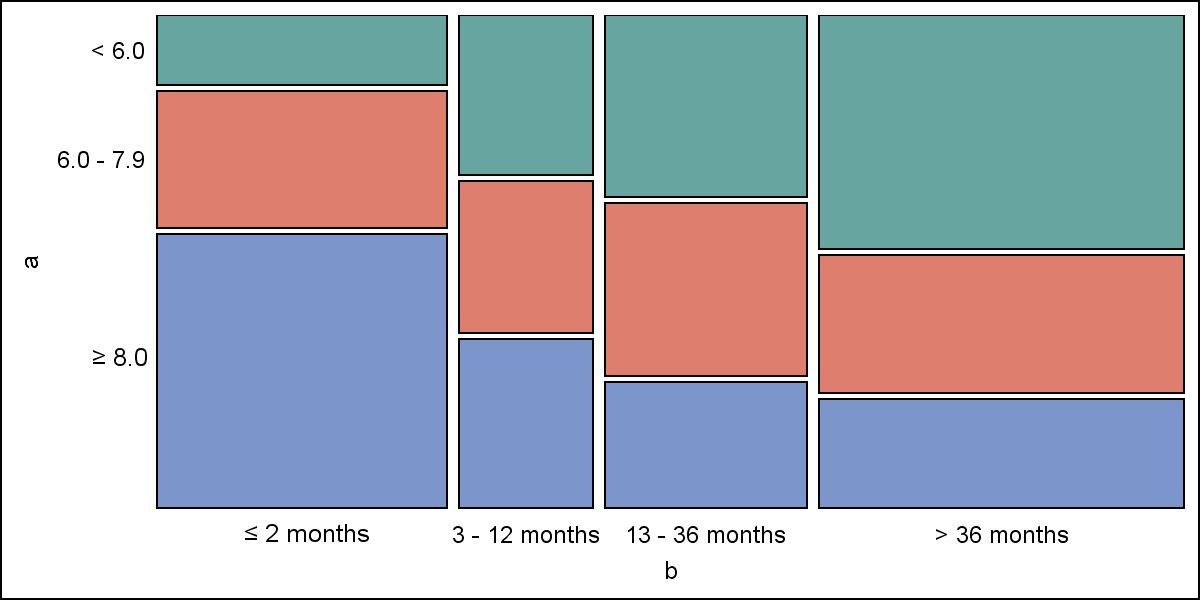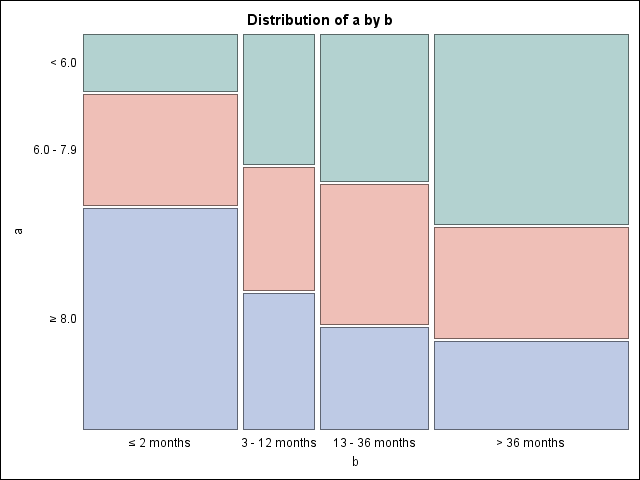## Special Characters using Format in ODS Graphics

I just upgraded to 9.3 and am finally getting around to learning ODS (Statistical) Graphics. For years now, I've been using ODS and routinely use escape characters in formats to get special characters into tables and text in HTML and PDF destinations. Now, however, when I try to add an ODS Statistical graph to my output, the special characters don't appear in the formats. Rather, the escape sequence displays. In the sample program below (9.3, Windows 7 32-bit, ODS Graphics on by default), the special characters (greater than or equal to, less than or equal to), which are encoded into formats, appear correctly in the table but not in the axis value labels of the mosaic plot. I tried it with both unicode characters and a font change, but neither works. I suspect I'm missing something obvious, and thanks in advance.

data one;
input a b c;
datalines;
1 1 36
1 2 38
1 3 65
1 4 151
2 1 70
2 2 36
2 3 62
2 4 89
3 1 140
3 2 40
3 3 45
3 4 70
;;;;
run;

proc format;
value af 1 = '< 6.0'
2 = '6.0 - 7.9'
3 = '(*ESC*){style [fontfamily=Symbol fontweight=bold]³} 8.0'
;
value bf 1 = '(*ESC*){style [fontfamily=Symbol fontweight=bold]£} 2 months'
2 = '3 - 12 months'
3 = '13 - 36 months'
4 = '> 36 months'
;
value a2f 1 = '< 6.0'
2 = '6.0 - 7.9'
3 = '(*ESC*){unicode ''2265''x} 8.0'
;
value b2f 1 = '(*ESC*){unicode ''2264''x} 2 months'
2 = '3 - 12 months'
3 = '13 - 36 months'
4 = '> 36 months'
;
quit;

proc freq;
table a * b / plots = mosaicplot;
weight c;
format a a2f. b b2f.;
run;

6 REPLIES 6AncaTilea
Pyrite | Level 9

## Re: Special Characters using Format in ODS Graphics

Karl...I am surprised nobody gave any answer to this reasonable question, as we are often faced with using special characters in the axis statements.

Well, I am not able to find the solution to your question, but I was able to find some work around...

So, first thing is that I can not get  the option 'mosaicplot' to work for me...do you have some other options turned on?

Anyway, I am instead using "freqplot"

See below:

proc freq;

table a * b / plots=freqplot(type=dot);*mosaicplot doesn't work for me;

weight c;

ods output crosstabFreqs = one_temp;

format a a2f. b b2f.;

run;

*delete any existing graphs from GSEG folder;

proc catalog c=work.gseg kill;

run; quit;

*format the V-axis as you wish;

goptions reset = all vsize = 4in hsize = 12;*divide the graphing window to 3 equal portions;

axis1 label = none value=(t=1 h = 1.5  f="Math" 'L' f="Arial" '2 months'

t=2 h = 1.5  f="Arial" '3 - 12 months'

t=3 h = 1.5  f="Arial" '13 - 36 months'

t=4  h = 1.5 f="Arial" '> 36 months')

minor = none;

*I plan to stack three plots, so, two of the plots need no H-axis -- see axis22, and the bottom plot needs the H-axis, see axis2;

axis2 label = none value = (f = "Arial" h = 2) order = 0 to 200 by 50;

axis22 label = none value = none minor = none ;

symbol v = dot h = 2 c = blue;

*this could be easily translated into a macro, but wanted to show to steps;

proc gplot data = one;where a = 1;

plot b*c/name = "a1" vaxis = axis1 haxis = axis22;

footnote1 move = (60,20) f= Arial h = 2 "a: < 6.0";

run;

quit;

proc gplot data = one;where a = 2;

plot b*c/name = "a2" vaxis = axis1 haxis = axis22;

footnote1 move = (60,23) f= Arial h = 2 "a: 6.0 - 7.9";

run;

quit;

proc gplot data = one;where a = 3;

plot b*c/name = "a3" vaxis = axis1 haxis = axis2;

footnote1 move = (60,23) f= Arial h = 2 "a: " h = 2 f = Math "M"  f = Arial h = 2 "8";

run;

quit;

filename plot "\\&your_path.\plot.jpeg";

goptions reset = all vsize = 12in hsize = 12 gsfname = plot gsfmode = replace device = jpeg;

proc greplay igout  = work.gseg

tc = sashelp.templt template = v3s

nofs nobyline;

treplay 1:a1 2:a2  3:a3;

run;quit;

Oh, I sure hope someone from SAS has a better way to handle special characters....## Re: Special Characters using Format in ODS Graphics

Thanks, Anca. Yeah I was kind of surprised I never heard anything either. In regard to the mosaicplot option not working for you, are you using 9.3? That proc freq option is new to 9.3 (I'm pretty sure), and, of course, ods graphics has to be turned on.

And, yes, I'm totally with you on your work-around. Inline formatting with escape sequences never worked with SAS/Graph, but SAS/Graph gives you all sorts of other ways--e.g., changing the font to symbol or wingdings or whatever, and good old Annotate--to get what you want, as you so clearly demonstrated. I was just hoping that ODS Graphics would honor ODS escape sequences. But I think it's clear that that's not the case. 9.4, maybe?

Thanks again.

KarlJay54
Meteorite | Level 14

## Re: Special Characters using Format in ODS Graphics

Mosaic plot was added to proc FREQ with SAS 9.3M2 or higher.

Supporting unicode characters throught data (or formats) needs a bit more work for ODS Graphics.  I have shown below how you can get what you want using GTL DRAW statements (sort of internal annotation).  This is not a satisfactory solution as it will only be good for a one off solution, and not scalable nor portable. You have to know where to place the text and if you add a title, it will change.

Code:

data one;
input a b c;
datalines;
1 1 36
1 2 38
1 3 65
1 4 151
2 1 70
2 2 36
2 3 62
2 4 89
3 1 140
3 2 40
3 3 45
3 4 70
;;;;
run;

proc format;
value af 1 = '< 6.0'
2 = '6.0 - 7.9'
3 = '(*ESC*){style [fontfamily=Symbol fontweight=bold]³} 8.0'
;
value bf 1 = '(*ESC*){style [fontfamily=Symbol fontweight=bold]£} 2 months'
2 = '3 - 12 months'
3 = '13 - 36 months'
4 = '> 36 months'
;
value a2f 1 = '< 6.0'
2 = '6.0 - 7.9'
3 = "a0"x
;
value b2f 1 = 'a0a0'x
2 = '3 - 12 months'
3 = '13 - 36 months'
4 = '> 36 months'
;
quit;

/*--Get mosaic plot data--*/
ods trace on;
ods output mosaicplot=mosaicplot;

proc freq;
table a * b / plots = mosaicplot;
weight c;
format a a2f. b b2f.;
run;

ods output close;
ods trace off;
proc print;run;

/*--Define Mosaic Plot Template--*/
proc template;
define statgraph mosaic;
begingraph;
layout region;
mosaicplotparm category=(_column _row) count=_freq / colorgroup=_row;
drawtext textattrs=(size=10pt) {unicode '2265'x} ' 8.0' / x=9 y=40;
drawtext textattrs=(size=10pt) {unicode '2264'x} ' 2 months' / x=25 y=9 width=20;
endlayout;
endgraph;
end;
run;

/*--Create Mosaic Plot--*/
ods listing image_dpi=200;
ods graphics / reset width=6in height=3in imagename='mosaic';
proc sgrender data=mosaicplot template=mosaic;
run;

Graph:Jay54
Meteorite | Level 14

## Re: Special Characters using Format in ODS Graphics

Recently, we have discovered a way to use UNICODE characters with a User Defined Format that works if you run the UNICODE version of SAS.  This is normally available under your SAS install in windows under "Additional Languages" folder in the All Programs->SAS.  No extra GTL coding or annotation is required.  Note:  Unicode characters are used in the format.

Here is the code:

/*--Run sas in unicode mode--*/

data one;
input a b c;
datalines;
1 1 36
1 2 38
1 3 65
1 4 151
2 1 70
2 2 36
2 3 62
2 4 89
3 1 140
3 2 40
3 3 45
3 4 70
;;;;
run;

proc format;
value af 1 = '< 6.0'
2 = '6.0 - 7.9'
3 = '≥ 8.0'
;
value bf 1 = '≤ 2 months'
2 = '3 - 12 months'
3 = '13 - 36 months'
4 = '> 36 months'
;

quit;

proc freq;
table a * b / plots = mosaicplot;
weight c;
format a af. b bf.;
run;

And the graph::AncaTilea
Pyrite | Level 9

Excellent!!!

Thank you!

## Re: Special Characters using Format in ODS Graphics

What do you know? I confess, I never even knew there was a Unicode version of SAS. I guess I missed that memo.

Do you know if that sort of thing will work with traditional SAS Graph as well? I haven't tried it; I'm just curious. If I had a dollar for every time I used "value = (tick1 = <change to a symbol font>special character)" in an Axis or a Legend statement, I'd be rich.

Thanks, Sanjay.

Karl

Discussion stats
• 6 replies
• 3307 views
• 1 like
• 3 in conversation